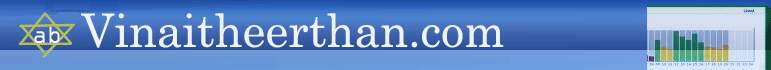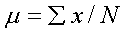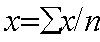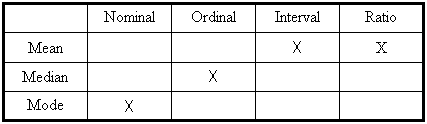# Tutorial on Introduction to biostatistics

Descriptive data analysis

The next stage of data analysis consists of descriptive and inferential data analysis. Descriptive data analysis provides the researcher a basic picture of the problem he is studying. It consists of:

§         Measures of Central Tendency

§         Measures of Skewness and Kurtosis

§         Measures of Dispersion.

### Measures of central tendency

A measure of central tendency is a value that represents a typical or central element of a data set. The important measures of central tendency are

a) Mean

b) Median

c) Mode

# Mean

Mean (average) is the sum of the data entries divided by the number of entries.Sample mean is denoted by    X   and the population mean is denoted by μ.

Population MeanWhere N is the number of items in the population

# Sample MeanWhere n is the number of items in the sample

Properties of Mean

•Data possessing an interval scale or a ratio scale, usually have a mean.

•All the values are included in computing the mean.

•A given set of data has a unique mean.

•The mean is affected by unusually large or small data values (known as outliers).

•The arithmetic mean is the only measure of central tendency where the sum of the deviations of each value from the mean is zero.

# Median

The median of a data set is the middle data entry when the data set is sorted in order.   If the data set contains an even number of elements, the median is the mean of the two middle entries. The median is the most appropriate measure of central tendency to use when the data under consideration are ranked data, rather than quantitative data

Mode

The mode of a data set is the entry that occurs with the greatest frequency.  A set may have no mode or may be bimodal when two entries each occur with the same greatest frequency. The mode is most useful when an important aspect of describing the data involves determining the number of times each value occurs.

If the data are qualitative then mode is particularly useful

The following table gives you an overview of which measure of central tendency is appropriate for each data measurement scale:Measures of Dispersion

Measures of Dispersion indicate the amount of variation or spread, in a data set.  There are four important measures of dispersion.

a)      Range

b)      Interquartile Range

c)      Variation

d)      Standard Deviation

a) The Range

The range is the difference between the largest and smallest observation.

The range is very sensitive to extreme values because it uses only the extreme values on each end of the ordered array. The range completely ignores the distribution of data.

The Interquartile Range

The interquartile range (midspread) is the difference between the

third and first quartiles.

Interquartile range         =          Q3        -           Q1

The interquartile range:
• Gives the range of the middle 50% of the data
• Is not affected by extreme values
• Ignores the distribution of data within the sample

Variance

The variance is the average of the squared differences between each observation and the mean.

Standard deviation

Standard deviation is the square root of the sample variance.

Properties of standard deviation

It lends itself to further mathematical analysis in a way that the range cannot because the standard deviation can be used in calculating other statistics.

It is worth noting that the standard deviation for nominal or ordinal data cannot be measured because it is not possible to calculate a mean for such data.

Relationships between two variables

Two of the important techniques used to study the relationship between two variables are correlation and regression.

Correlation

·        Measures association between two variables

·        In graph form it would be shown as a ‘scatter diagram’ putting the scores for one variable on the horizontal X axis and the values for the other variable on the vertical Y axis.

·        The pattern shows the strength of the association between the two variables and also whether it is a ‘positive’ or ‘negative’ relationship.

·         A ‘positive’ relationship means that as the value on one variable increases so does the value on the other variable.

·        A ‘negative’ relationship means that as the value on one variable increases, the value on the other variable decreases.

# Measures of correlation

There are two measures of correlation. One is Pearson’s product- moment correlation - r and other is Spearman’s rank order co-efficient – rho. Both the measures will tell us only how closely the two variables are connected but they cannot tell us whether one causes the other. Correlation values can range from –1 to +1

Interpretation of correlation value

Equal to 0                                 no correlation

Less than .2                              is very low

Between .2 and .4                   is low

Between .41 and .70                is moderate

Between .71 and .90                is high

Over .91                                  is very high

Equal to 1                                 perfect correlation

# Scatter diagrams can also be used to depict the correlation between two variables. The greater the spread/scatter, the less will bethe correlation value. The following scatter diagram shows the correlation between age and weight in a cancer study.From merely inspecting the diagram we can infer that there is low correlation because the spread is large while the location of the scatter plot towards the upper right tells us that whatever correlation may exist is likely to be positive. The Pearson correlation coefficient for the same data was determined to be 0.196, which confirms a very low positive correlation.

# Properties of correlation

• Correlation will not establish a cause and effect relationship
• Correlation may sometimes be a non-sense correlation
• It is very sensitive to extreme values

Simple Regression analysis:

It gives the equation of a straight line and enables prediction of one variable value from the other. Normally, the dependent variable is plotted on the Y axis and the independent variable on the X axis. There are 3 major assumptions - first, any value of x and y are normally distributed. Second, the variability of y should be the same for each value of y. Third; the relationship between the two variables is linear.

The equation of a regression line is: “y=a + bx”  where ‘a’ is the intercept, ‘b’ is the slope, ‘x’ is the independent variable and ‘y’ is the dependent variable. The slope ‘b’ is sometimes called regression coefficient and it has the same sign as correlation co-efficient (i.e., ‘r’).

Probability

Probability is defined as the likelihood of an event or outcome in trial.

p(A) =    Number of outcomes classified as A

Total number of possible outcomes